User:IssaRice/Computability and logic/List of possibilities for completeness and decidability

Yes Yes Yes Smith's example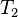$T_2$.
Yes Yes No Smith's example of$T_1$ with just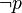$\neg p$ as an axiom inside a propositional logic with propositional atoms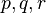$p,q,r$.
Yes No Yes Assuming the theory is effectively axiomatized and consistent, this is not possible. To see why, suppose the theory is negation-complete. We could then computably enumerate all the theorems (since the theory is effectively axiomatized), and after a finite amount of time we will find exactly one of$\phi$ or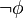$\neg \phi$, which gives us an algorithm for deciding whether or not$\phi$ is a theorem.
No Yes Yes First-order logic with two binary predicates$R$ and$S$ with the axiom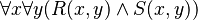$\forall x \forall y (R(x,y)\wedge S(x,y))$.
No Yes No i think we can mimic$T_1$ by defining some predicate such that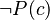$\neg P(c)$ is an axiom, with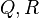$Q,R$ as additional predicates.
No No Yes Assuming the theory is effectively axiomatized and consistent, this is not possible. To see why, suppose the theory is negation-complete. We could then computably enumerate all the theorems (since the theory is effectively axiomatized), and after a finite amount of time we will find exactly one of$\phi$ or$\neg \phi$, which gives us an algorithm for deciding whether or not$\phi$ is a theorem.